Question

# A particle leaves the origin with an initial velocity v⃗ =(2.40m/s)x^, and moves with constant acceleration...

A particle leaves the origin with an initial velocity v⃗ =(2.40m/s)x^, and moves with constant acceleration a⃗ =(−1.90m/s2)x^+(3.20m/s2)y^.

How far does the particle move in the x direction before turning around?

What is the particle's velocity at this time?

Calculate the particle's position at t = 0.500 s, 1.00 s, 1.50 s, and 2.00 s. Use these results to sketch x and y positions versus time for the particle.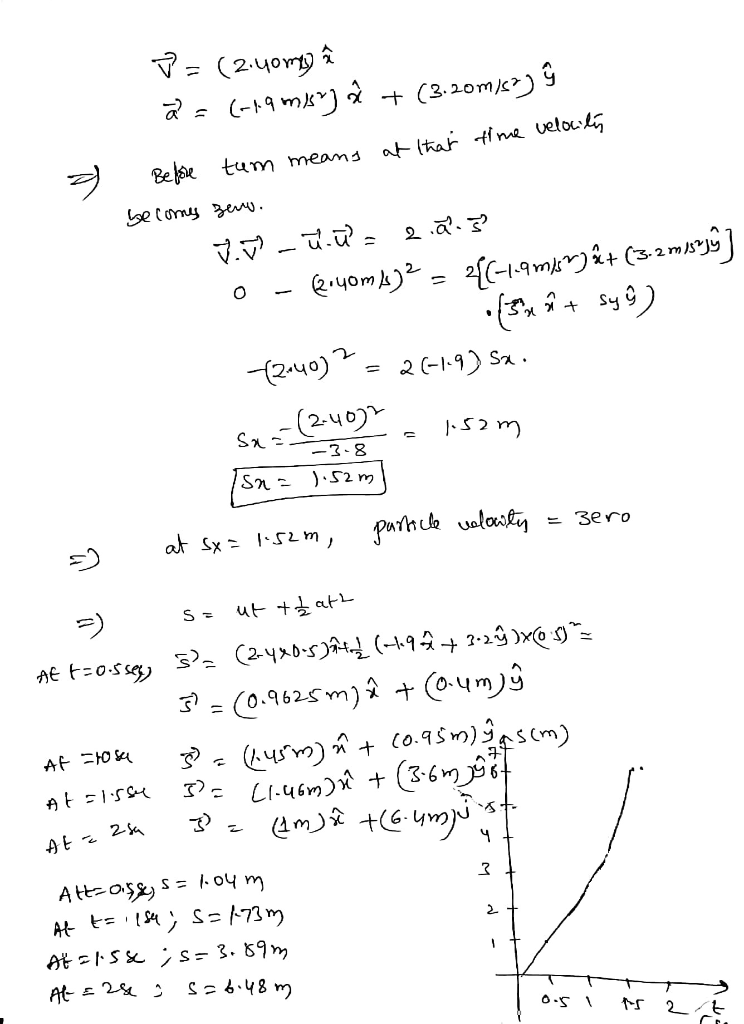#### Earn Coins

Coins can be redeemed for fabulous gifts.

Similar Homework Help Questions
• ### Course Contents »> > Problem Set 3Timer Notes Evaluate Feedback Print Info particle leaves the origin...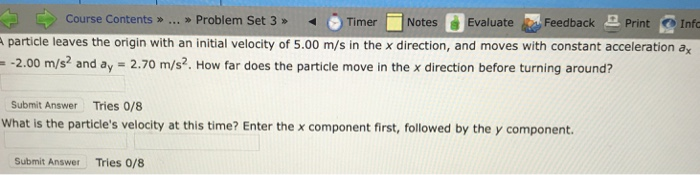Course Contents »> > Problem Set 3Timer Notes Evaluate Feedback Print Info particle leaves the origin with an initial velocity of 5.00 m/s in the x direction, and moves with constant acceleration a -2.00 m/s and ay 2.70 m/s2. How far does the particle move in the x direction before turning around? Submit Answer Tries O/8 What is the particle's velocity at this time? Enter the x component first, followed by the y component. Submit Answer Tries 0/8

• ### A particle leaves the origin with an initial velocity = (5.18) m/s and a constant acceleration =...

A particle leaves the origin with an initial velocity = (5.18) m/s and a constant acceleration = ( - 4.85 - 4.80) m/s2. When the particle reaches its maximum xcoordinate, what are (a) its velocity, (b) its position vector?

• ### At t = 0, a particle leaves the origin with a velocity of 9.0 m/s in the positive y direction and moves in the xy plane with a constant acceleration of (2.0i - 4.0j)m/s2

At t = 0, a particle leaves the origin with a velocity of 9.0 m/s in the positive y direction and moves in the xy plane with a constant acceleration of (2.0i - 4.0j)m/s2. At the instant the x coordinate of the particle is 15 m, what is the speed of the particle?  10 m/s  16 m/s  12 m/s  14 m/s  26 m/s

• ### A particle leaves the origin with an initial velocity of v-([vO])i m/s where vO-8.25 and a...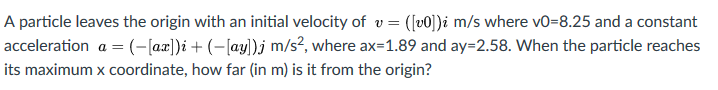A particle leaves the origin with an initial velocity of v-([vO])i m/s where vO-8.25 and a constant acceleration a = (-[az])i + (-lay)j m/s2, where ax-1.89 and ay-258. When the particle reaches its maximum x coordinate, how far (in m) is it from the origin?

• ### A particle leaves the origin with an initial velocity = (6.93) = 6.931 m/s and a...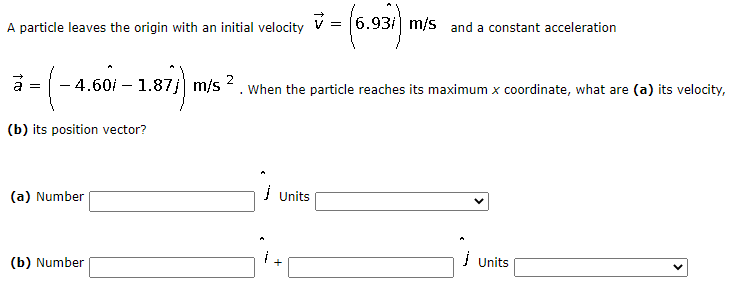A particle leaves the origin with an initial velocity = (6.93) = 6.931 m/s and a constant acceleration (-- - 4.601 – 1.87j m/s2 When the particle reaches its maximum x coordinate, what are (a) its velocity, (b) its position vector? (a) Number Units (b) Number + Units

• ### A particle starts from the origin at t Owith a velocity of 6.2j and moves in...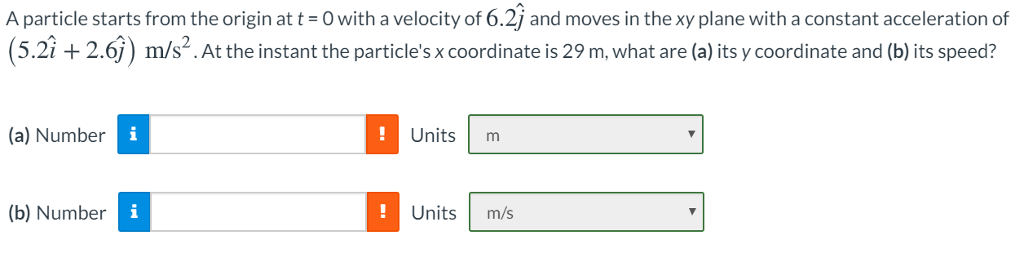A particle starts from the origin at t Owith a velocity of 6.2j and moves in the xy plane with a constant acceleration of (5.2i +2.6j) m/s2. At the instant the particle's x coordinate is 29 m, what are (a) its y coordinate and (b) its speed? (a) Number Unitsm (b) Number Units m/s

• ### At t = 0, a particle leaves the origin with a velocity of 9 0 m/s...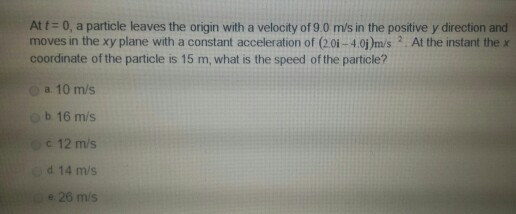At t = 0, a particle leaves the origin with a velocity of 9 0 m/s in the positive y direction and moves in the xy plane with a constant acceleration of (2.0i - 4.0j)m/s^2. At the instant the x coordinate of the particle is 15 m, what is the speed of the particle? 10m/S 16 m/s 12 m/s 14 m/s 26 m/s

• ### In the figure, particle A moves along the line y = 33 m with a constant velocity v of magnitude 2.7 m/s and directed parallel to the x axis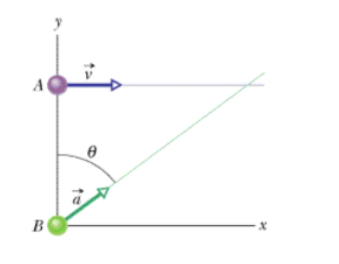In the figure, particle A moves along the line y = 33 m with a constant velocity v of magnitude 2.7 m/s and directed parallel to the x axis. At the instant particle A passes the y axis, particle B leaves the origin with zero initial speed and constant acceleration ã of magnitude 0.46 m/s2. What angle θ between a and the positive direction of the y axis would result in a collision?

• ### At t = 0, a particle moving in the xy plane with constant acceleration has a...

At t = 0, a particle moving in the xy plane with constant acceleration has a velocity of vector v i = (3.00 i - 2.00 j) m/s and is at the origin. At t = 3.70 s, the particle's velocity is vector v = (7.40 i + 6.90 j) m/s. (Use the following as necessary: t. Round your coefficients to two decimal places.) (a) Find the acceleration of the particle at any time t. vector a = m/s2 (b)...

• ### At t = 0, a particle moving in the xy plane with constant acceleration has a...

At t = 0, a particle moving in the xy plane with constant acceleration has a velocity of vector v i = (3.00 i - 2.00 j) m/s and is at the origin. At t = 3.60 s, the particle's velocity is vector v = (8.90 i + 7.70 j) m/s. (Use the following as necessary: t. Round your coefficients to two decimal places.) (a) Find the acceleration of the particle at any time t. vector a = m/s2 (b)...